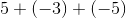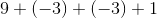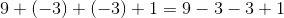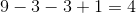## Example Questions

### Example Question #1 : How To Add Negative Numbers

Add:Explanation:

Anytime a negative number is added, it is similar to subtraction.  Recovert the expression to the correct form.  Simplify.The correct answer is.

### Example Question #2 : How To Add Negative Numbers

Add the negative numbers:Explanation:

In order to add the negative numbers, we need to eliminate the double signs and the parentheses.  A positive and a negative sign will result in a negative sign.Evaluate the terms on the right.The answer is:### Example Question #14 : Negative Numbers

a, b, c are integers.

abc < 0

ab > 0

bc > 0

Which of the following must be true?

a + b < 0

ac < 0

a > 0

b > 0

a – b > 0

a + b < 0

Explanation:

Let's reductively consider what this data tells us.

Consider each group (a,b,c) as a group of signs.

From abc < 0, we know that the following are possible:

(–, +, +), (+, –, +), (+, +, –), (–, –, –)

From ab > 0, we know that we must eliminate (–, +, +) and (+, –, +)

From bc > 0, we know that we must eliminate (+, +, –)

Therefore, any of our answers must hold for (–, –, –)

This eliminates immediately a > 0, b > 0

Likewise, it eliminates a – b > 0 because we do not know the relative sizes of a and b. This could therefore be positive or negative.

Finally, ac is a product of negatives and is therefore positive. Hence ac < 0 does not hold.

We are left with a + b < 0, which is true, for two negatives added must be negative.